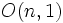# Lorentzian manifold

This article defines a differential manifold with the following additional structure -- the structure group is reduced to: Lorentzian group viz a group of the form$O(n,1)$

## Definition

A Lorentzian manifold is the following data:

• A differential manifold$M$
• A symmetric nondegenerate bilinear form of type$(n,1)$ on each tangent space, such that the form varies smoothly with the point.

In other words, a Lorentzian manifold is the reduction of the structure group of a differential manifold to the Lorentzian group$O(n,1)$.

Lorentzian manifolds are special cases of pseudo-Riemannian manifolds. Thus, all the generic constructs for a pseudo-Riemannian manifold apply to Lorentzian manifolds.